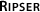# Table 2 Overview of existing software for the computation of PH that have an accompanying peer-reviewed publication (and also, because of its performance)

Software javaPlex Perseus jHoles Dionysus PHAT DIPHA Gudhi SimpPers Ripser
(a) Language Java C++ Java C++ C++ C++ C++ C++ C++
(b) Algorithms for PH standard, dual, zigzag Morse reductions, standard standard (uses javaPlex) standard, dual, zigzag standard, dual, twist, chunk, spectral sequence twist, dual, distributed dual, multifield simplicial map twist, dual
(c) Coefficient field $$\mathbb{Q}$$, $$\mathbb{F}_{p}$$ $$\mathbb{F}_{2}$$ $$\mathbb{F}_{2}$$ $$\mathbb{F}_{2}$$ (standard, zigzag), $$\mathbb{F}_{p}$$ (dual) $$\mathbb{F}_{2}$$ $$\mathbb{F}_{2}$$ $$\mathbb{F}_{p}$$ $$\mathbb{F}_{2}$$ $$\mathbb{F}_{p}$$
(d) Homology simplicial, cellular simplicial, cubical simplicial simplicial simplicial, cubical simplicial, cubical simplicial, cubical simplicial simplicial
(e) Filtrations computed VR, W, $$\mathrm{W}_{\nu}$$ VR, lower star of cubical complex WRCF VR, α, C̆ - VR, lower star of cubical complex VR, α, W, lower star of cubical complex - VR
(f) Filtrations as input simplicial complex, zigzag, CW simplicial complex, cubical complex - simplicial complex, zigzag boundary matrix of simplicial complex boundary matrix of simplicial complex - map of simplicial complexes -
(g) Additional features Computes some homological algebra constructions, homology generators weighted points for VR - vineyards, circle-valued functions, homology generators - - - - -
(h) Visualization barcodes persistence diagram - - - persistence diagram - - -
1. The symbol ‘-’ signifies that the associated feature is not implemented. For each software package, we indicate the following items. (a) The language in which it is implemented. (b) The implemented algorithms for the computation of barcodes from the boundary matrix. (c) The coefficient fields for which PH is computed, where the letter p denotes any prime number in the coefficient field $$\mathbb{F}_{p}$$. (d) The type of homology computed. (e) The filtered complexes that are computed, where VR stands for Vietoris–Rips complex, W stands for the weak witness complex, $$\mathrm{W}_{\nu}$$ stands for parametrized witness complexes, WRCF stands for the weight rank clique filtration, α stands for the alpha complex, and Č for the Čech complex. Perseus, DIPHA, and Gudhi implement the computation of the lower-star filtration  of a weighted cubical complex; one inputs data in the form of a d-dimensional array; the data is then interpreted as a d-dimensional cubical complex, and its lower-star filtration is computed. (See the Tutorial in Additional file 2 of the SI, for more details.) Note that DIPHA and Gudhi use the efficient representation of cubical complexes presented in , so the size of the cubical complex that is computed by these libraries is smaller than the size of the resulting complex with Perseus. (f) The filtered complexes that one can give as input. javaPlex supports the input of a filtered CW complex for the computation of cellular homology ; in contrast with simplicial complexes, there do not currently exist algorithms to assign a cell complex to point-cloud data. (g) Additional features implemented by the library. javaPlex supports the computation of some constructions from homological algebra (see  for details), and Perseus implements the computation of PH with the VR for points with different ‘birth times’ (see Section 5.1.3). The library Dionysus implements the computation of vineyards  and circle-valued functions . Both javaPlex and Dionysus support the output of representatives of homology classes for the intervals in a barcode. (h) Whether visualization of the output is provided